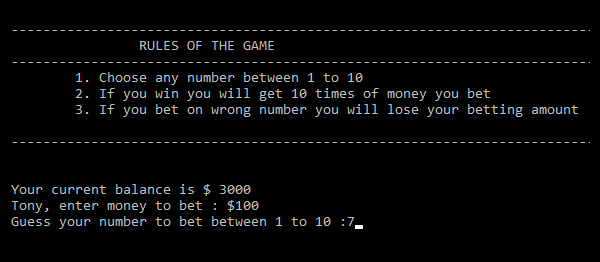CASINO NUMBER GUESSER GAME IN C++ WITH SOURCE CODE

This is a simple c++ project. In this project, you just have to guess the winning number. If you can place your bet with a certain amount of money. This project contains a lot of field validations to the project. To run this project you can use Dev C++ or...

Type : Project

File Size : 434.2 KBSimilar Projects and Reports

CASINO NUMBER GUESSER GAME IN C++ WITH SOURCE CODE

This is a simple c++ project. In this project, you just have to guess the winning number. If you can place your bet with a certain amount of money. This project contains a lot of field validations to the project. To run this project you can use Dev C++ or...

GUESS NUMBER IN PYTHON WITH SOURCE CODE

The Guess Number In Python is a simple project developed using Python. This project is a command based which creates random number by taking the number from the users input. This project is an interesting and simple project. The project is not completely ...

Guess Number In JavaScript With Source Code

The Guess Number is a simple project developed using HTML5, CSS, and JavaScript. This game is about guessing the randomly generated number. It is a fun educational game that challenges kids to find a number based on greater than or less than feedback. If ...

Number List In C++ With Source Code

The Number List is a simple project developed using c++ programming language. The project adds and stores a list of numbers as the basic work. Here, the user can add as many numbers as they want and calculate the mean of the total, also find the smallest ...

Guess Game In Python With Source Code

The Guess Game In Python is a simple project developed using Python. This project is an interesting and simple project. This project is a command-based which generates a random number between 1 and 50. And the player has to guess that random number to win...

Math Game In C++ With Source Code

The Math Game is a single-player game. This project is an interesting project. Here, the user can test their calculation skills through this app. If you are able to give the right solution to the problem, then you will get a score of 10 on each question. ...